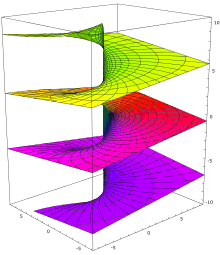# Principal branch of the log function

• I
I'm learning complex analysis right now, and I'm reading from Joseph Taylor's Complex Variables.

On Theorem 1.4.8, it says "If a log is the branch of the log function determined by an interval I, then log agrees with the ordinary natural log function on the positive real numbers if and only if the interval I contains 0."

Can anyone help me understand what this means? Is it just saying that the properties of the real log function only hold if the interval I contains 0? I don't understand why.

## Answers and Replies

fresh_42
Mentor(from: https://de.wikipedia.org/wiki/Logarithmus#Komplexer_Logarithmus; engl.: https://en.wikipedia.org/wiki/Logarithm#Complex_logarithm)

You see, that the logarithm ##w## for a value ##z##, i.e. ##e^w=z## has all solutions ##w+2k\pi i\; , \;k\in\mathbb{Z}##. To make it unique, we have to choose one of these branches, i.e. one value for ##k##. This prinicpal value is usually determined by ##- \pi < \operatorname{Im}(w) \leq \pi\,.## Of course we could also say ##-\pi/2 < \operatorname{Im}(w) \leq 3/2 \pi## or any other interval with ##0## in it. But if we climb up, such that zero isn't part of the interval anymore, then we are in the complex world, i.e. we ran around the circle once before regarding the logarithm, but this "run around once" has no real counterpart, because in the reals we would arrive at where we started, whereas in the complexes we climbed up a branch, which is not identical to where we started.